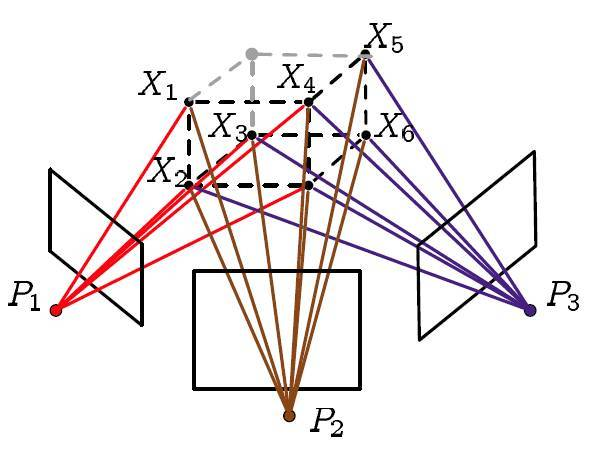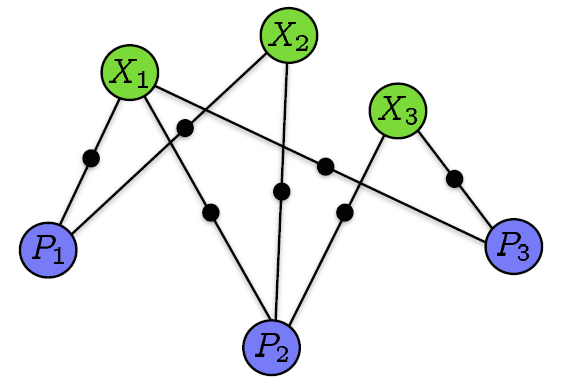Bundle Adjustment简述 | HyperPlane

• 射影相机几何模型
• 对极几何
• 凸优化
• 矩阵理论

## 这才是真的开始

Bundle Adjustment（之后在不引起歧义的情况下用BA代替，你问为什么？笔者懒啊-。-），大概似乎也许好像有近百年的历史了吧（没错，可以称为state-of-art的视觉SLAM在几年前才用上将近上百岁的算法），中文译为光束法平差，大概大家看到更多的翻译可能为束调整捆集调整或者捆绑调整等等。这么多翻译笔者最喜欢的还是光束法平差，一看就比其它的更专业逼格更高嘛，其它的翻译都太直译了。当然最重要的是光束法平差完美的表达了BA的来源、原理和计算过程，而其他的只是强调了将很多数据放在一起进行优化计算这个事。不信？那我们来分析一下嘛。

## BA模型到底是怎么来的？• 其实第一次投影指的就是相机在拍照的时候三维空间点投影到图像上
• 然后我们利用这些图像对一些特征点进行三角定位（triangulation，很多地方翻译为三角化或者三角剖分等等，当然笔者最喜欢的还是三角定位，显然是利用几何信息构建三角形来确定三维空间点的位置嘛，相关内容请参考对极几何）
• 最后利用我们计算得到的三维点的坐标（注意不是真实的）和我们计算得到的相机矩阵（当然也不是真实的）进行第二次投影，也就是重投影

## 进入数学模式！$e_{ij}=u_{ij}-v_{ij}$

BA是要将所有重投影误差的和最小化，那么这里自然就要开始求和了。

$\min_{R_i,t_i,X_j} \sum_{i,j} \sigma_{ij}\|u_{ij}-v_{ij}\|_2$

## 接下来就应该开始计算了！

$\mathbf{f(x)} = \sum_{i,j} \sigma_{ij}\|u_{ij}-v_{ij}\| _2^2$

### 最速下降法

$x_k = x_{k-1}-\lambda \nabla \mathbf{f(x_{k-1})}$

### Newton型方法

Newton型方法也就是这种思想，首先将函数利用泰勒展开到二次项：

$\mathbf{f(x + \delta x) \approx \varphi(\delta x) = f(x) + \nabla f(x)\delta x + \frac{1}{2}\delta x^T H(x) \delta x}$

$\mathbf{H}$为Hessian矩阵，是二次偏导矩阵。

$\mathbf{\varphi'(\delta x) = \nabla f(x) + H \delta x = 0 }$

$\Longrightarrow \mathbf{ \delta x = -H^{-1}\nabla f(x) }$

Newton型方法收敛的时候特别快，尤其是对于二次函数而言一步就可以得到结果。但是该方法有个最大的缺点就是Hessian矩阵计算实在是太复杂了，并且Newton型方法的迭代并不像最速下降法一样保证每次迭代都是下降的。

### Gauss-Newton方法

\begin{aligned} \mathbf{f(x + \delta x)} & \approx \mathbf{\| e + J(x) \delta x \|} _2^2\\ & = \mathbf{\delta x^T J^T J \delta x + 2e^T J \delta x + e^Te} \end{aligned}

$\mathbf{J^{T} J \delta x = - J^{T} e}$

$\mathbf{x = x + \delta x}$

$\mathbf{\nabla f(x)} = 2\mathbf{J^Te}$

Gauss-Newton方法就避免了求Hessian矩阵，并且在收敛的时候依旧很快。但是依旧无法保证每次迭代的时候函数都是下降的（虽然从上式可以推导出来是下降方向，但是步长可能过长）。

### LM方法

LM方法就是在以上方法基础上的改进，通过参数的调整使得优化能在最速下降法和Gauss-Newton法之间自由的切换，在保证下降的同时也能保证快速收敛。

Gauss-Newton最后需要求解的方程为

$\mathbf{J^{T} J \delta x = - J^{T} e}$

LM算法在此基础上做了更改，变成了

$\mathbf{(J^{T} J + \lambda I) \delta x = - J^{T} e}$

$\mathbf{\lambda I \delta x = - J^T e}$

$\Longrightarrow \mathbf{\delta x = - \lambda^{-1}J^T e}$

$\mathbf{(J^{T} J + \lambda diag(J^T J)) \delta x = - J^{T} e}$

LM算法就由此保证了每次迭代都是下降的，并且可以快速收敛。

## 还没完呢！别忘了还要解方程

LM算法主体就是一个方程的求解，也是其计算量最大的部分。当其近似于最速下降法的时候没有什么好讨论的，但是当其近似于Gauss-Newton法的时候，这个最小二乘解的问题就该好好讨论一下了。以下的讨论就利用Gauss-Newton的形式来求解。

### 稀疏矩阵的Cholesky分解

$A \approx R^T R$

$R^TRx = b$

$x = R^{-1}R^{-T}b$

### 为什么说我们的矩阵是稀疏的

$\mathbf{J} = \left[ \begin{array}{cc|cc} \frac{\partial f}{\partial P_1} & & & \frac{\partial f}{\partial X_2} \\\\ & \frac{\partial f}{\partial P_2} & \frac{\partial f}{\partial X_1} & \\\\ & \frac{\partial f}{\partial P_2} & & \frac{\partial f}{\partial X_2} \end{array} \right]$

### 开始计算吧！

$\mathbf{J^{T} J \delta x = - J^{T} e}$

$\mathbf{J}=[A|B]$

$[A|B]^T[A|B]\mathbf{\delta x}=-[A|B]^T e$

$\begin{bmatrix} A^TA & A^TB \\\\ B^TA & B^TB \end{bmatrix} \begin{bmatrix} \mathbf{\delta x}_A \\\\ \mathbf{\delta x}_B \end{bmatrix} = - \begin{bmatrix} A^T e \\\\ B^T e \end{bmatrix}$

$\begin{bmatrix} U & W \\\\ W^T & V \end{bmatrix} \begin{bmatrix} \mathbf{\delta x}_A \\\\ \mathbf{\delta x}_B \end{bmatrix} = \begin{bmatrix} e _A \\\\ e _B \end{bmatrix}$

$\begin{bmatrix} I & -WV^{-1} \\\\ 0 & I \end{bmatrix} \begin{bmatrix} U & W \\\\ W^T & V \end{bmatrix} \begin{bmatrix} \mathbf{\delta x}_A \\\\ \mathbf{\delta x}_B \end{bmatrix} = \begin{bmatrix} I & -WV^{-1} \\\\ 0 & I \end{bmatrix} \begin{bmatrix} e _A \\\\ e _B \end{bmatrix}$

$\begin{bmatrix} U-WV^{-1}W^T & 0 \\\\ W^T & V \end{bmatrix} \begin{bmatrix} \mathbf{\delta x}_A \\\\ \mathbf{\delta x}_B \end{bmatrix} = \begin{bmatrix} e _ A - WV^{-1}e _B \\\\ e _B \end{bmatrix}$

$\begin{cases} (U-WV^{-1}W^T) \delta _A = e _ A - WV^{-1}e _B \\\\ W^T \delta _A + V \delta _B = e _B \end{cases}$

## 注意事项！

### 协方差矩阵

$\mathbf{J^{T} J \delta x = - J^{T} e}$

$\mathbf{J^{T} \Sigma_x^{-1} J \delta x = - J^{T} \Sigma_x^{-1} e}$

## 参考文献

• Triggs B, McLauchlan P F, Hartley R I, et al. Bundle adjustment—a modern synthesis[C]//International workshop on vision algorithms. Springer Berlin Heidelberg, 1999: 298-372.
• Hartley R, Zisserman A. Multiple view geometry in computer vision[M]. Cambridge university press, 2003.
• Barfoot T D. STATE ESTIMATION FOR ROBOTICS[J]. 2017.